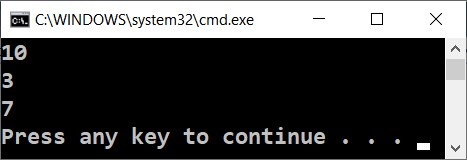# Arithmetic Operations

Let's examine the basic arithmetic operations in programming. We can add, subtract, multiply and divide numbers using the operators +, -, * and /.

## Video: Arithmetic Operators

Watch a video lesson about the arithmetic operators: https://youtu.be/XOtEuEUbA4M.

## Summing up Numbers: Operator +

We can sum up numbers using the + operator:

var a = 5;
var b = 7;
var sum = a + b; // the result is 12


## Subtracting Numbers: Operator -

Subtracting numbers is done using the - operator:

var a = int.Parse(Console.ReadLine());
var result = a - b;
Console.WriteLine(result);


Here is the result of the execution of this program (with numbers 10 and 3):## Multiplying Numbers: Operator *

For multiplication of numbers we use the * operator:

var a = 5;
var b = 7;
var product = a * b; // 35


## Dividing Numbers: Operator /

Dividing numbers is done using the / operator. It works differently with integers and floating point numbers.

• When we divide two integers, an integer division is applied, and the obtained output is without its fractional part. Example: 11 / 3 = 3.
• When we divide two numbers and at least one of them is a float number, a floating division is applied, and the obtained result is a float number, just like in math. Example 11 / 4.0 = 2.75. When it cannot be done with exact precision, the result is being rounded, for example 11.0 / 3 = 3.66666666666667.
• The integer division by 0 causes an exception during runtime (runtime exception).
• Float numbers divided by 0 do not cause an exception and the result is +/- infinity or a special value NaN. Example 5 / 0.0 = ∞.

Here are a few examples with the division operator:

var a = 25;
var i = a / 4;      // we are applying an integer division:
// the result of this operation will be 6 – the fractional part will be cut,
// because we are dividing integers
var f = a / 4.0;    // 6.25 – floating division. We have set the number 4 to be interpreted
// as a float by adding a decimal separator followed by zero
var error = a / 0;  // Error: Integer divided by zero


### Dividing Integers

Let's examine a few examples for integer division (remember that when we divide integers in C# the result is an integer):

var a = 25;
Console.WriteLine(a / 4);  // Integer result: 6
Console.WriteLine(a / 0);  // Error: divide by 0


### Dividing Floating-Point Numbers

Let's look at a few examples for floating division. When we divide floating point numbers, the result is always a float number and the division never fails, and works correctly with the special values +∞ and -∞:

var a = 15;
Console.WriteLine(a / 2.0);   // Float result: 7.5
Console.WriteLine(a / 0.0);   // Result: Infinity
Console.WriteLine(-a / 0.0);  // Result: -Infinity
Console.WriteLine(0.0 / 0.0); // Result: NaN (Not a Number), e.g. the result
// from the operation is not a valid numeric value


When printing the values and -∞, the console output may be ?, because the console in Windows does not work correctly with Unicode and breaks most of the non-standard symbols, letters and special characters. The example above would most probably give the following result:

7.5
?
-?
NaN